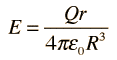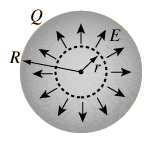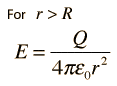# Magnitude of an Electric Field of a Sphere at a Distance

• Redfire66
In summary, the equation for the electric field inside a sphere is E = KeQ/r^2. The field is the same outside the sphere as it is for a point charge of the same total charge located at the sphere's centre.

## Homework Statement

So I was given the equation that in a sphere, if at a distance r outisde a sphere of radius R (The sphere insulated, I guess it matters since it would prevent charge to leave?)
The electric field E = kqR/r^3
I'm not sure how they got this

## Homework Equations

Flux = Integral of E *delta A
Flux = total charge/epsilon0
(Density was used which I ended up using to go in circles)
p = q/V

## The Attempt at a Solution

So I started off, please correct me if I get anything wrong
Flux = integral of E deltaA = E*4piR^2
I think it should use the full radius of the sphere here. But I hope you can explain if this is correct and why
Then Flux is also equal to q/epsilon0
q = p/V where V = 4/3 pi*r^3

In the end I ended up with E*4piR^2 = p4/3(pi)r^3/epsilon
Then when I look at the answer it shows that r^3 is on the bottom so I'm kind of stuck here.
I'd appreciate it if you could explain how this all works instead of just giving a solution with no explanation

Last edited:
Redfire66 said:
in a sphere, if at a distance r inside a sphere
This is about a field inside a uniformly charged sphere, with no other charges inside the sphere? That'll be zero. Or did you mean outside the sphere, at distance r from the sphere's centre?

Sorry I was thinking about something else; yes I meant outside the sphere where r > R

Redfire66 said:
E = kqR/r^3
Doesn't look right to me. Outside the sphere, the field is equivalent to a point charge of the same total charge placed at the sphere's centre. The radius of the sphere is irrelevant there.
Redfire66 said:
Flux = integral of E deltaA = E*4piR^2
You need something representing charge density in there. What is the E on the right hand side?

hang on ... for some reason I assumed hollow spherical shell. Maybe you mean a solid uniformly charged sphere. In that case there is a field inside, but it is proportional to r. Outside, it's still the same as for a point charge.

Dear Red,

This could also be a notation issue. ##\vec E## is a vector. It has a magnitude and a direction, just like a force or a velocity. The magnitude of the field due to a point charge is $$E ={ kq\over r^2}$$The direction is away from the charge (if positive). This is often written as$$\vec E ={ kq\over r^2}\hat r$$ ##\hat r## is the unit vector in the radial direction. ##r^2## is distance squared, a scalar. Other authors use the notation$$\vec E ={ kq\over r^3}\vec r\qquad {\text or}\qquad \vec E ={ kq\over |\vec r|^3}\vec r\$$so there you see a third power popping up, but effectively it's reduced to a square by the r in the numerator.

The result for a massive sphere instead of a point charge is found by using spherical symmetry and Gauss's theorem. The nice outcome is that outside the sphere the fields are exactly the same !

•Redfire66
Thanks for the reply. This is starting to make sense to me. However when I'm reading through my textbook it states that if the electric field is outside the sphere (r > R) then the field is written as
E = KeQ/r^2
However if r < R, meaning inside of a sphere the field would be E = KeQr/R^3
I can derive it d solve for this second equation using the integral for volume and area and such.. but I don't quite understand it. Would you mind explaining this? I assume it's because I don't understand how this equation is formulated or the theory behind it that I can't get the grasp on this.

Last edited:
Redfire66 said:
However if r < R, meaning inside of a sphere the field would be E = KeQr/R^3
I can derive it d solve for this second equation using the integral for volume and area and such.. but I don't quite understand it. Would you mind explaining this? I assume it's because I don't understand how this equation is formulated or the theory behind it that I can't get the grasp on this.
I'm not quite sure what you are saying there. Are you saying you can derive this formula, but don't understand why it's right at some conceptual level, or that you do not see how to obtain it?
If the latter, consider the sphere in two parts - the region at radius < r and the region at radius between r and R.
As noted, the field due to the region at radius <r is the same as for a point charge of the same total charge as that region, located at the sphere's centre. What is the total charge of that portion, and what field does it produce at radius r?
For the region at radius > r, as noted, there is no field generated inside a uniformly charged spherical shell. The region at radius >r can be thought of as nested spherical shells from radius r to radius R.

This link is where I saw it
http://hyperphysics.phy-astr.gsu.edu/hbase/electric/elesph.html#c3
Also my textbook showed me how to derive it, so I do know how to derive the formula but I don't understand why it's right.
"For a radius r < R, a Gaussian surface will enclose less than the total charge and the electric field will be less. Inside the sphere of charge, the field is given by:" (second last box in the link) and is followed by an equation shows that it is r/R^3. And since r and R are different, I don't see how there is a connection between the radius of the sphere and the the electric field at the point r.

Redfire66 said:
This link is where I saw it
http://hyperphysics.phy-astr.gsu.edu/hbase/electric/elesph.html#c3
Also my textbook showed me how to derive it, so I do know how to derive the formula but I don't understand why it's right.
"For a radius r < R, a Gaussian surface will enclose less than the total charge and the electric field will be less. Inside the sphere of charge, the field is given by:" (second last box in the link) and is followed by an equation shows that it is r/R^3. And since r and R are different, I don't see how there is a connection between the radius of the sphere and the the electric field at the point r.
It follows from the fact that for a uniformly charged spherical shell of total charge Q:
1. there is no field inside the shell
2. the field outside the shell is the same as for a point charge Q located at the centre of the sphere.
If you accept/understand that, consider your solid sphere radius R as composed of concentric shells.
For those shells of radius a < r, apply rule 2. R and r will both feature in the answer because the total charge for that subset depends on the ratio between them, i.e. as ##Qr^3R^{-3}##. Since we're looking at the field distance r from the centre, we get the form ##Qr^3R^{-3}r^{-2} = QrR^{-3}##.
For those shells outside radius r apply rule 1. They generate no field at radius r.

Redfire66 said:

## Homework Statement

So I was given the equation that in a sphere, if at a distance r outisde a sphere of radius R (The sphere insulated, I guess it matters since it would prevent charge to leave?)
The electric field E = kqR/r^3
This last line unfortunately put your kind helpers on the wrong track (by switching r and R). No big deal.

It became clear to me when, using the link in post #9, the best I could find wasfor the case(both pictures probably ## \copyright ## CSU)
And the correct hyperphysics tekst (idem (c) CSU) is

In the last expression, the sphere radius R is in the denominator.
The distance from the center for which the field is evaluated is r.

Outside the sphere the field goes like 1/r2So that at r = R there is continuity.

And it all makes sense.

Just so that no confusion remains: Haruspex makes things a little complicated in post #10:
What he writes is correct, but might confuse casual readers (like me) who don't directly
distinguish shell from sphere.

For a uniformly charged spherical shell there is no contribution to the electric field inside that shell

There is an electric field inside a uniformly charged solid non-conducting sphere. We've just discussed it.

And finally, there is no electric field inside a charged solid conducting sphere. The charges would simply go with the field until they couldn't go any further: they repel each other and sit on the surface. See this page on the same website.

Last edited:
Redfire66 said:
Thanks for the reply. This is starting to make sense to me. However when I'm reading through my textbook it states that if the electric field is outside the sphere (r > R) then the field is written as
E = KeQ/r^2
However if r < R, meaning inside of a sphere the field would be E = KeQr/R^3
I can derive it d solve for this second equation using the integral for volume and area and such.. but I don't quite understand it. Would you mind explaining this? I assume it's because I don't understand how this equation is formulated or the theory behind it that I can't get the grasp on this.

Redfire66 said:
This link is where I saw it
http://hyperphysics.phy-astr.gsu.edu/hbase/electric/elesph.html#c3
Also my textbook showed me how to derive it, so I do know how to derive the formula but I don't understand why it's right.
"For a radius r < R, a Gaussian surface will enclose less than the total charge and the electric field will be less. Inside the sphere of charge, the field is given by:" (second last box in the link) and is followed by an equation shows that it is r/R^3. And since r and R are different, I don't see how there is a connection between the radius of the sphere and the the electric field at the point r.

Re the appearance of R in the equation for E when r < R :

The only reason R appears here is that the total charge of the sphere is given. So for 0 to R It is converted to a charge density by dividing by ##{4\over 3} \pi R^3## and then to a charge for 0 to r by multiplying with ##{4\over 3}\pi r^3##. Then Gauss's law is applied.

From Gauss's law you can conclude that the charge in the range r to R does not contribute to the field at distance r from the center. It's understandable that this is a bit strange. One way to make it plausible to yourself is to do the integration, but it's hard work, I think.

BvU said:So that at r = R there is continuity.
Thanks I do understand now how the equation works out if r < R of the sphere.. however now it makes more sense to me than if the Gaussian radius is outside the sphere.
Can you explain a bit more simply put why r = R if r > R? It doesn't seem right since I always thought the further away you are from a charge the weaker the field.
Edit:
So let's say we're trying to solve for the field when it's outside the sphere.
so r > R
then the integral of EdA = ke*q
Then E = ke*q/(4pi*epsilon*r^2)
That's what I did.
However if I work it out using the density then I would have r/R^3.

Last edited:
However if I work it out using the density then I would have r/R^3
Could you post how you come to this result ?

So let r be the radius of the Gaussian sphere
And I have r < R. When R is the sphere of the ball.
If I take the integral I have E∫dA = q/ε
Then ∫dA = 4*pi*r^2
and p = qV1...... V1 in this case would be the volume of the sphere
E (4pi*r^2) = pV/ε
E = p(4/3)(pi*r^3)/[ε(4pi*r^2)]
and we know that p = the density, which can be written as Q/V2.... V2 would be the sphere's volume
And then after cancelling things out I end up with E = Qr/[(4pi*ε*R^3)] = KeQr/R^3
This is what I did when the area was LESS than the actual radius of the sphere. NOT when it was greater than.
But wouldn't this be the same as if r > R? Or maybe I'm missing some sort of concept that I should've known...
Or did I do something wrong? I understand that from post 10 that there is no field inside, however I don't understand what that has to do with this equation.

Last edited:
Oh I see now, after thinking about it a bit, if the there is no charge inside then I can just take into account the Gaussian area and that would be the radius hence which you stated that r = R right? Then the equation would then cancel out and I would have KeQ/R^2 where R is the radius of the actual area I am given! This would make sense, however I'd just like to confirm it with you if that's true.

Can you explain a bit more simply put why r = R if r > R? It doesn't seem right since I always thought the further away you are from a charge the weaker the field.
First of all: I sure hope I never stated that (it's a contradiction). But I do sense some hesitation in the r>R case. Difficult to rephrase without falling into repetiton.
I can give it a try, though I suspect you already have it right. In itself the r>R calculation is by far the simplest, but the fuzz from the calculation for r<R seems to seep through into the r>R calculation. (Am I totally wrong ?).

What is difficult in this treatise is the "double role" small r plays for
the field inside the sphere:
• on the one hand for the ##Q'## expression for the charge inside the Gaussian volume (leading via ##\rho## to ##\rho {4\over 3} \pi r^3## ) and
• on the other hand for the ##\int dA## expression of the area of the surface of that same Gaussian volume (## 4\pi r^2##)
The ##Q'## ends up in the numerator, the area in the denominator for the E formula. Result: one factor r in the E formula. You worked that out very well.

The "only reason" the R comes into that formula is because we "want" an expression in Q, not in ##\rho##. ( from ##\rho = \displaystyle {Q\over {4\over 3}\pi R^3})##.

For the field outside the sphere you could in fact do the same integration for the charge inside the Gaussian volume: ##\displaystyle \int_V \rho dV \;=\; \int _0^r \rho \;4\pi r^2 dr##, only this time ##\rho## is only ##\ne## 0 up to r=R, so the integral 'stops' at r=R and you get ##\rho V## which is equal to Q.
And the integration for the surface area still gives (## 4\pi r^2##).

I did a search for r = R in the thread and the only time I used it was to illustrate that the two formulas for E give the same result at that radius. So I need a better pointer or quote to focus on a possible problematic passage in the thread...

Alright, that makes sense to what I also thought. Thanks for your help